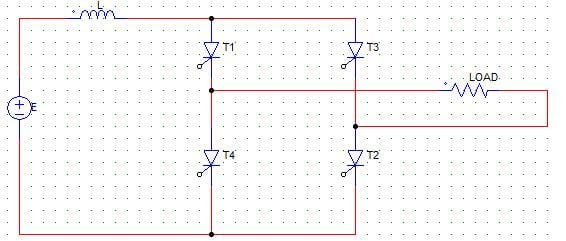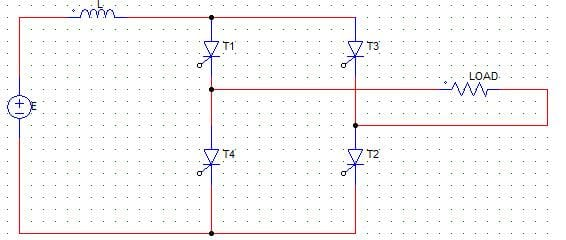Test: Current Source Inverters

# Test: Current Source Inverters

Test Description

## 10 Questions MCQ Test Power Electronics | Test: Current Source Inverters

Test: Current Source Inverters for Electrical Engineering (EE) 2023 is part of Power Electronics preparation. The Test: Current Source Inverters questions and answers have been prepared according to the Electrical Engineering (EE) exam syllabus.The Test: Current Source Inverters MCQs are made for Electrical Engineering (EE) 2023 Exam. Find important definitions, questions, notes, meanings, examples, exercises, MCQs and online tests for Test: Current Source Inverters below.
Solutions of Test: Current Source Inverters questions in English are available as part of our Power Electronics for Electrical Engineering (EE) & Test: Current Source Inverters solutions in Hindi for Power Electronics course. Download more important topics, notes, lectures and mock test series for Electrical Engineering (EE) Exam by signing up for free. Attempt Test: Current Source Inverters | 10 questions in 30 minutes | Mock test for Electrical Engineering (EE) preparation | Free important questions MCQ to study Power Electronics for Electrical Engineering (EE) Exam | Download free PDF with solutions
 1 Crore+ students have signed up on EduRev. Have you?
Test: Current Source Inverters - Question 1

### In voltage source inverters (VSIs), the amplitude of the output voltage is

Detailed Solution for Test: Current Source Inverters - Question 1

In VSIs the input voltage is maintained at a constant value and the amplitude of the output voltage does not depend on the load conditions. However, the waveform of the load current as well as its magnitude depends upon the nature of the load impedance.

Test: Current Source Inverters - Question 2

### In current source inverters (CSIs)

Detailed Solution for Test: Current Source Inverters - Question 2

In CSIs, the amplitude of the output current is independent on the load impedance, as the input current (to the CSI) is kept constant.

Test: Current Source Inverters - Question 3

### In current source inverters

Detailed Solution for Test: Current Source Inverters - Question 3

In order that the current input to the CSI must remain ripple free an constant, L-filter is used before the CSI (in series with the energy source).

Test: Current Source Inverters - Question 4

In the below shown, the ideal single-phase CSI circuit has constant input source current of I amps. The output current waveform varies fromDetailed Solution for Test: Current Source Inverters - Question 4

Considering the SCRs as ideal voltage sources, when T1, T2 are on the load current is = I. When T3, T4 conduct, the load current is negative with respect to the previous value and = -I.

Test: Current Source Inverters - Question 5

In a 3-phase VSI operating in square-wave mode, the output line voltage is free from

Detailed Solution for Test: Current Source Inverters - Question 5

The line voltage is the difference of two phase voltages, the 3rd harmonic gets cancelled out, though it is present in the phase voltages.

Test: Current Source Inverters - Question 6

In voltage source inverters (VSIs), the output currents _____________

Detailed Solution for Test: Current Source Inverters - Question 6

In VSIs the input voltage is maintained at a constant value and the amplitude of the output voltage does not depend on the load conditions. However, the waveform of the load current as well as its magnitude depends upon the nature of the load impedance.

Test: Current Source Inverters - Question 7

In current source inverters (CSIs), the output voltage’s

Detailed Solution for Test: Current Source Inverters - Question 7

In CSIs, the amplitude of the output current is independent on the load impedance, as the input current (to the CSI) is kept constant. However, the magnitude of output voltage and its waveform depends upon the nature of the load impedance.

Test: Current Source Inverters - Question 8

A CSI converters

Detailed Solution for Test: Current Source Inverters - Question 8

CSI converts the input dc current to an ac current at its output terminals.

Test: Current Source Inverters - Question 9

T1, T2 are triggered at 0 and T3, T4 at T/2. If both the pairs of SCRs conduct for equal duration and the load consists of a capacitor. ThenDetailed Solution for Test: Current Source Inverters - Question 9

The load current waveform is a square wave with frequency = 1/T. As the load consists of a capacitor, dv/dt must be constant over every half cycle. This slope is positive from zero to T/2 and negative from T/2 to T.

Test: Current Source Inverters - Question 10

Force-commutated CSIs need

Detailed Solution for Test: Current Source Inverters - Question 10

All the CSIs need capacitors for their commutation if force commutation is required. Force commutation is essential for lagging power factors.

## Power Electronics

5 videos|39 docs|63 tests
 Use Code STAYHOME200 and get INR 200 additional OFF Use Coupon Code
Information about Test: Current Source Inverters Page
In this test you can find the Exam questions for Test: Current Source Inverters solved & explained in the simplest way possible. Besides giving Questions and answers for Test: Current Source Inverters, EduRev gives you an ample number of Online tests for practice

## Power Electronics

5 videos|39 docs|63 tests